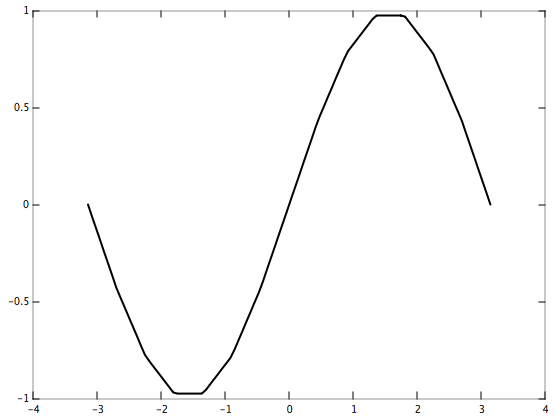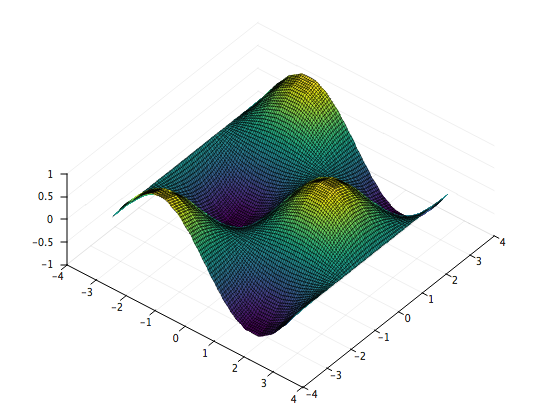I made a header-only C++ library for arbitrary dimension linear interpolation (a.k.a. multilinear interpolation). The design philosophy is to push as much to compile-time as possible by template metaprogramming.

Instructions for how to include it in your work are on the GitHub project page.

Below are some simple examples of its usage.

## Examples

### 1d

Let’s interpolate y = sin(x) on the interval [-pi, pi] using 15 evenly-spaced data points.

using namespace mlinterp;

// Boundaries of the interval [-pi, pi]
constexpr double b = 3.14159265358979323846, a = -b;

// Subdivide the interval [-pi, pi] using 15 evenly-spaced points and
// evaluate sin(x) at each of those points
constexpr int nxd = 15, nd[] = { nxd };
double xd[nxd];
double yd[nxd];
for(int n = 0; n < nxd; ++n) {
xd[n] = a + (b - a) / (nxd - 1) * n;
yd[n] = sin(xd[n]);
}

// Subdivide the interval [-pi, pi] using 100 evenly-spaced points
// (these are the points at which we interpolate)
constexpr int ni = 100;
double xi[ni];
for(int n = 0; n < ni; ++n) {
xi[n] = a + (b - a) / (ni - 1) * n;
}

// Perform the interpolation
double yi[ni]; // Result is stored in this buffer
interp(
nd, ni, // Number of points
yd, yi, // Output axis (y)
xd, xi  // Input axis (x)
);

// Print the interpolated values
cout << scientific << setprecision(8) << showpos;
for(int n = 0; n < ni; ++n) {
cout << xi[n] << "\t" << yi[n] << endl;
}Note that the points do not have to be evenly spaced. Try modifying the above to use a non-uniform grid!

### 2d

Let’s interpolate z = sin(x)cos(y) on the interval [-pi, pi] X [-pi, pi] using 15 evenly-spaced points along the x axis and 15 evenly-spaced points along the y axis.

using namespace mlinterp;

// Boundaries of the interval [-pi, pi]
constexpr double b = 3.14159265358979323846, a = -b;

// Discretize the set [-pi, pi] X [-pi, pi] using 15 evenly-spaced
// points along the x axis and 15 evenly-spaced points along the y axis
// and evaluate sin(x)cos(y) at each of those points
constexpr int nxd = 15, nyd = 15, nd[] = { nxd, nyd };
double xd[nxd];
for(int i = 0; i < nxd; ++i) {
xd[i] = a + (b - a) / (nxd - 1) * i;
}
double yd[nyd];
for(int j = 0; j < nyd; ++j) {
yd[j] = a + (b - a) / (nyd - 1) * j;
}
double zd[nxd * nyd];
for(int i = 0; i < nxd; ++i) {
for(int j = 0; j < nyd; ++j) {
const int n = j + i * nyd;
zd[n] = sin(xd[i]) * cos(yd[j]);
}
}

// Subdivide the set [-pi, pi] X [-pi, pi] using 100 evenly-spaced
// points along the x axis and 100 evenly-spaced points along the y axis
// (these are the points at which we interpolate)
constexpr int m = 100, ni = m * m;
double xi[ni];
double yi[ni];
for(int i = 0; i < m; ++i) {
for(int j = 0; j < m; ++j) {
const int n = j + i * m;
xi[n] = a + (b - a) / (m - 1) * i;
yi[n] = a + (b - a) / (m - 1) * j;
}
}

// Perform the interpolation
double zi[ni]; // Result is stored in this buffer
interp(
nd, ni,        // Number of points
zd, zi,        // Output axis (z)
xd, xi, yd, yi // Input axes (x and y)
);

// Print the interpolated values
cout << scientific << setprecision(8) << showpos;
for(int n = 0; n < ni; ++n) {
cout << xi[n] << "\t" << yi[n] << "\t" << zi[n] << endl;
}Note that the x and y axes do not have to be identical: they can each have any number of unequally spaced points. Try modifying the above to use different x and y axes!

## Higher dimensions (3d, 4d, …)

In general, if you have k dimensions with axes x1, x2, …, xk, the interp routine is called as follows:

interp(
nd,  ni,                          // Number of points
yd,  yi,                          // Output axis
x1d, x1i, x2d, x2i, ..., xkd, xki // Input axes
);